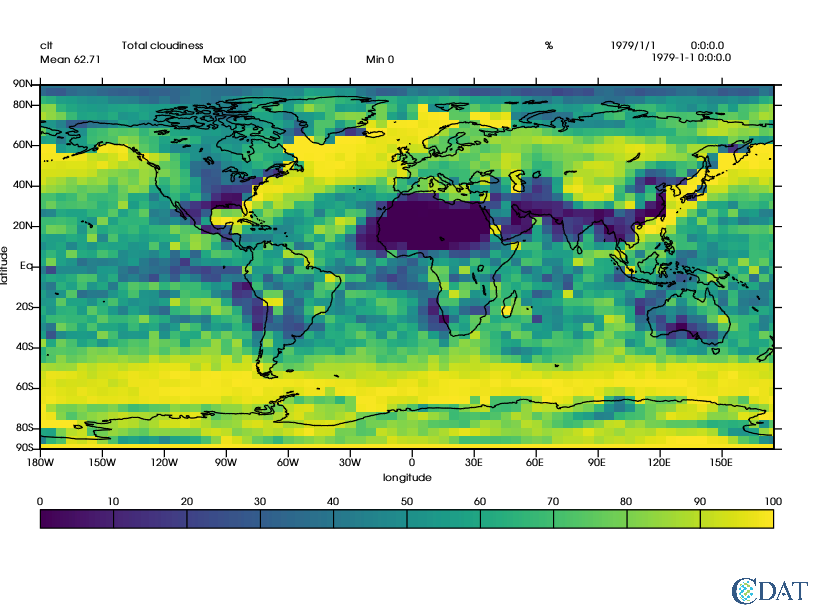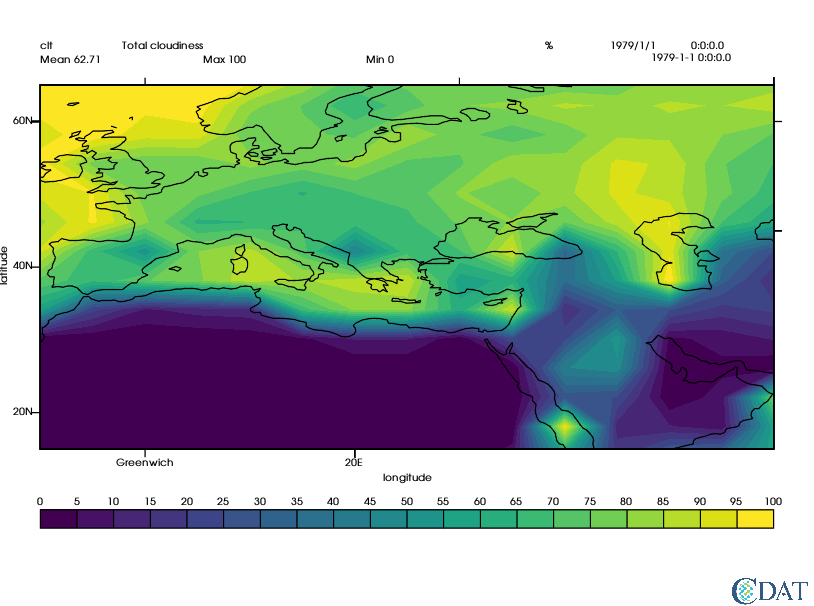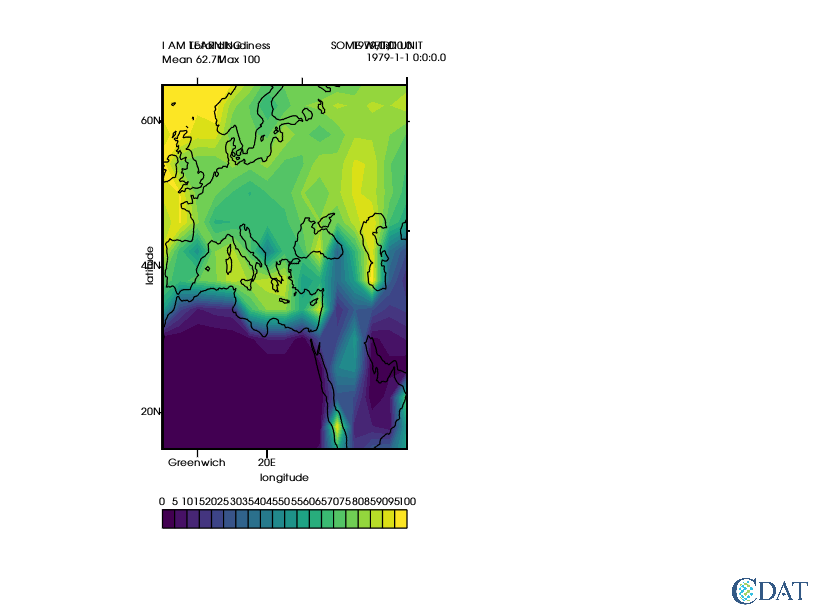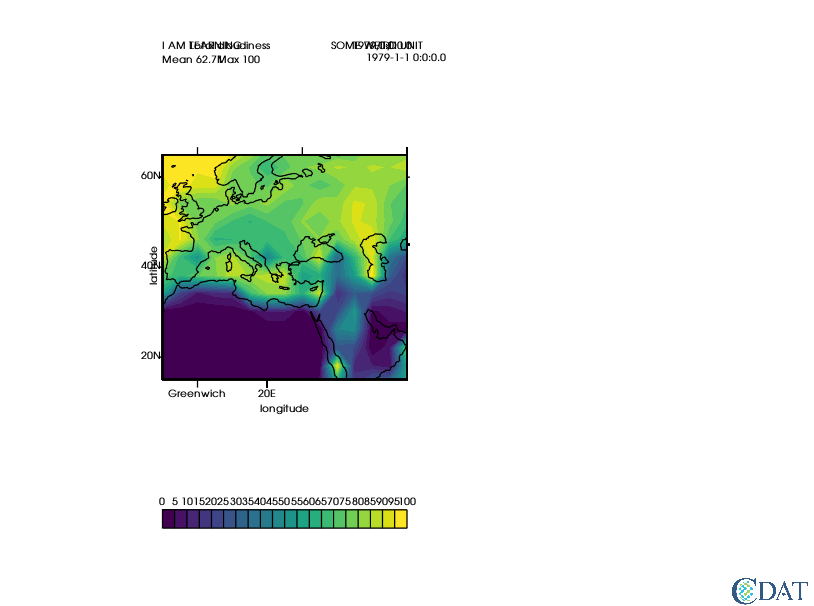# VCS Example¶

This tutorial provides an example, from start to finish, of downloading some data and plotting it.

The CDAT software was developed by LLNL. This tutorial was written by Charles Doutriaux. This work was performed under the auspices of the U.S. Department of Energy by Lawrence Livermore National Laboratory under Contract DE-AC52-07NA27344.

Download the Jupyter Notebook

In :
import vcs
import cdms2


# Download Data¶

Back to Top

Download some sample data.

In :
vcs.download_sample_data_files()

Downloading: 'th_yr.nc' from 'https://cdat.llnl.gov/cdat/sample_data/' in: /Users/davis278/miniconda3/envs/nightly_mesa/share/cdat/sample_data/th_yr.nc
Downloading: 'th_yr.nc' from 'https://cdat.llnl.gov/cdat/sample_data/' in: /Users/davis278/miniconda3/envs/nightly_mesa/share/cdat/sample_data/th_yr.nc
Downloading: 'th_yr.nc' from 'https://cdat.llnl.gov/cdat/sample_data/' in: /Users/davis278/miniconda3/envs/nightly_mesa/share/cdat/sample_data/th_yr.nc


# Open Data File¶

Open the clt.nc NetCDF file which contains total cloudiness data and assign that open file to the variable f. The file contains three types of data:

• clt: total cloudiness on a monthly basis for 10 years from January 1979 up to and including December 1988.
• u: eastward component of wind speed (i.e. wind blowing from west to east)
• v: northward component of wind speed (i.e. wind blowing from south to north)
In :
f=cdms2.open(vcs.sample_data+"/clt.nc")


# Load Data into a Variable¶

We'll load the total cloudiness data, clt, into the s variable (for sample data).

In :
s=f("clt")


# Set Up VCS for Plotting¶

Next we'll create a vcs canvas called x which is basically a window in which to display the data.

Setting bg=True means the data will be plotted in the background (bg) meaning the data will plot in this notebook and not in a separate window on your computer.

In :
x=vcs.init(bg=True)


# Plot the Data¶

First we'll plot the data just as it is using the default settings in VCS, which in this case creates a boxfill plot (the default plotting style if two dimensional or higher data is present).

In :
x.plot(s)

Out:## Explore Other Graphic Methods¶

First we'll list all the graphic methods available.

In :
vcs.listelements()

Out:
['1d',
'3d_dual_scalar',
'3d_scalar',
'3d_vector',
'boxfill',
'colormap',
'display',
'fillarea',
'font',
'fontNumber',
'format',
'isofill',
'isoline',
'line',
'list',
'marker',
'meshfill',
'projection',
'scatter',
'streamline',
'taylordiagram',
'template',
'textcombined',
'textorientation',
'texttable',
'vector',
'xvsy',
'xyvsy',
'yxvsx']

### Isofill¶

Let's create an isofill object called gm (for graphic method) using the createisofill method.

In :
gm = vcs.createisofill()


Let's see what options available for manipulating the gm object that uses the isofill graphics method.

In :
gm.list()

---------- Isofill (Gfi) member (attribute) listings ----------
graphics method = Gfi
name = __isofill_808490450874473
projection = linear
xticlabels1 = *
xticlabels2 = *
xmtics1 =
xmtics2 =
yticlabels1 = *
yticlabels2 = *
ymtics1 =
ymtics2 =
datawc_x1 = 1e+20
datawc_y1 =  1e+20
datawc_x2 =  1e+20
datawc_y2 =  1e+20
datawc_timeunits =  days since 2000
datawc_calendar =  135441
xaxisconvert =  linear
yaxisconvert =  linear
missing =  (0.0, 0.0, 0.0, 100.0)
ext_1 =  False
ext_2 =  False
fillareastyle =  solid
fillareaindices =  
fillareacolors =  
fillareaopacity =  []
fillareapixelspacing =  None
fillareapixelscale =  None
levels =  ([1.0000000200408773e+20, 1.0000000200408773e+20],)
legend =  None


The graphic method controls how things are drawn:

• datawc stands for dataworldcoordinates.
• x/yticlabels are the labels to use on the x/y axes (python dictionaries {location_value:"string"}.
• x/ymtics are the ticks that do not have strings/labels attached.
• missing is color to use for misssing values (index in colormap, (r,g,b,o) or "string").
• fillarea are the contour properties.
In :
gm.datawc_x1 = -10
gm.datawc_x2 = 60
gm.datawc_y1= 15
gm.datawc_y2 = 65

gm.xticlabels1 = {0:"Greenwich", 20:"20E"}
gm.yticlabels2 = {0:"Equator",60:"Arctic Circle", 45:"45N"}

levels = list(range(0,101,5)) # specify the iso contours to use
colors = vcs.getcolors(levels) # automatically picks colors that will be spread across the range of your data.
gm.levels = levels
gm.fillareacolors = colors

x.clear()
x.plot(s,gm,bg=True)

Out:# Control Location of Plot Elements¶

To control the location of elements, like the plot title, we use a template.

In :
t = vcs.createtemplate()


t.list() lists all the properties that can be set.

In :
t.list()

---------- Template (P) member (attribute) listings ----------
method = P
name = __template_563570891808101
orientation = 0
member =  file
priority = 1
x = 0.0500000007451
y = 0.0130000002682
texttable = default
textorientation = default
member =  function
priority = 1
x = 0.0500000007451
y = 0.0130000002682
texttable = default
textorientation = default
member =  logicalmask
priority = 1
x = 0.0500000007451
y = 0.0329999998212
texttable = default
textorientation = default
member =  transformation
priority = 1
x = 0.0500000007451
y = 0.0529999993742
texttable = default
textorientation = default
member =  source
priority = 1
x = 0.0500000007451
y = 0.941999971867
texttable = default
textorientation = default
member =  dataname
priority = 1
x = 0.0500000007451
y = 0.922999978065
texttable = default
textorientation = default
member =  title
priority = 1
x = 0.15000000596
y = 0.922999978065
texttable = default
textorientation = default
member =  units
priority = 1
x = 0.670000016689
y = 0.922999978065
texttable = default
textorientation = default
member =  crdate
priority = 1
x = 0.75
y = 0.922999978065
texttable = default
textorientation = default
member =  crtime
priority = 1
x = 0.850000023842
y = 0.922999978065
texttable = default
textorientation = default
member =  comment1
priority = 1
x = 0.10000000149
y = 0.954999983311
texttable = default
textorientation = default
member =  comment2
priority = 1
x = 0.10000000149
y = 0.975000023842
texttable = default
textorientation = default
member =  comment3
priority = 1
x = 0.10000000149
y = 0.995000004768
texttable = default
textorientation = default
member =  comment4
priority = 1
x = 0.10000000149
y = 0.999000012875
texttable = default
textorientation = default
member =  xname
priority = 1
x = 0.5
y = 0.21
texttable = default
textorientation = defcenter
member =  yname
priority = 1
x = 0.006
y = 0.56
texttable = default
textorientation = defup
member =  zname
priority = 0
x = 0.0
y = 0.995000004768
texttable = default
textorientation = default
member =  tname
priority = 0
x = 0.0
y = 0.995000004768
texttable = default
textorientation = default
member =  xunits
priority = 0
x = 0.600000023842
y = 0.21
texttable = default
textorientation = default
member =  yunits
priority = 0
x = 0.006
y = 0.658999979496
texttable = default
textorientation = defup
member =  zunits
priority = 0
x = 0.0
y = 0.995000004768
texttable = default
textorientation = default
member =  tunits
priority = 0
x = 0.0
y = 0.995000004768
texttable = default
textorientation = default
member =  xvalue
priority = 0
x = 0.800000011921
y = 0.941999971867
format = default
texttable = default
textorientation = default
member =  yvalue
priority = 0
x = 0.800000011921
y = 0.922999978065
format = default
texttable = default
textorientation = default
member =  zvalue
priority = 1
x = 0.800000011921
y = 0.902999997139
format = default
texttable = default
textorientation = default
member =  tvalue
priority = 0
x = 0.800000011921
y = 0.883000016212
format = default
texttable = default
textorientation = default
member =  mean
priority = 1
x = 0.0500000007451
y = 0.899999976158
format = default
texttable = default
textorientation = default
member =  min
priority = 1
x = 0.449999988079
y = 0.899999976158
format = default
texttable = default
textorientation = default
member =  max
priority = 1
x = 0.25
y = 0.899999976158
format = default
texttable = default
textorientation = default
member =  xtic1
priority = 1
y1 = 0.259999990463
y2 = 0.24699999392
line = default
member =  xtic2
priority = 1
y1 = 0.860000014305
y2 = 0.871999979019
line = default
member =  xmintic1
priority = 0
y1 = 0.259999990463
y2 = 0.248999999285
line = default
member =  xmintic2
priority = 0
y1 = 0.860000014305
y2 = 0.868000014305
line = default
member =  ytic1
priority = 1
x1 = 0.0500000007451
x2 = 0.0399999991059
line = default
member =  ytic2
priority = 1
x1 = 0.949999988079
x2 = 0.959999978542
line = default
member =  ymintic1
priority = 0
x1 = 0.0500000007451
x2 = 0.0450000017881
line = default
member =  ymintic2
priority = 0
x1 = 0.949999988079
x2 = 0.954999983311
line = default
member =  xlabel1
priority = 1
y = 0.234999999404
texttable = default
textorientation = defcenter
member =  xlabel2
priority = 0
y = 0.880000004768
texttable = default
textorientation = defcenter
member =  ylabel1
priority = 1
x = 0.0399999991059
texttable = default
textorientation = defright
member =  ylabel2
priority = 0
x = 0.959999978542
texttable = default
textorientation = default
member =  box1
priority = 1
x1 = 0.0500000007451
y1 = 0.259999990463
x2 = 0.949999988079
y2 = 0.860000014305
line = default
member =  box2
priority = 0
x1 = 0.0
y1 = 0.300000011921
x2 = 0.920000016689
y2 = 0.879999995232
line = default
member =  box3
priority = 0
x1 = 0.0
y1 = 0.319999992847
x2 = 0.910000026226
y2 = 0.860000014305
line = default
member =  box4
priority = 0
x1 = 0.0
y1 = 0.0
x2 = 0.0
y2 = 0.0
line = default
member =  line1
priority = 0
x1 = 0.0500000007451
y1 = 0.560000002384
x2 = 0.949999988079
y2 = 0.560000002384
line = default
member =  line2
priority = 0
x1 = 0.5
y1 = 0.259999990463
x2 = 0.5
y2 = 0.860000014305
line = default
member =  line3
priority = 0
x1 = 0.0
y1 = 0.52999997139
x2 = 0.899999976158
y2 = 0.52999997139
line = default
member =  line4
priority = 0
x1 = 0.0
y1 = 0.990000009537
x2 = 0.899999976158
y2 = 0.990000009537
line = default
member =  legend
priority = 1
x1 = 0.0500000007451
y1 = 0.129999995232
x2 = 0.949999988079
y2 = 0.159999996424
line = default
texttable = default
textorientation = defcenter
offset = 0.01
member =  data
priority = 1
x1 = 0.0500000007451
y1 = 0.259999990463
x2 = 0.949999988079
y2 = 0.860000014305


The most commonly used elements/members are: dataname, title, mean, max, min, units, data, and legend.

• data controls the area where to plot the data.
• legend is the area used by the legend/colorbar.

The values come from the data plotted.

Most common attributes are:

• x/y coordinate are in % of page.
• priority is the order of drawing with 2 drawing above 1 and 3 drawing above 2. A value of 0 turns the priority value off.

See below for more information on text objects, but they are basically controlled via textorientation and texttable objects properties.

In :
t.min.priority =0 # turn off min
s.id = "I AM LEARNING" # change dataname
s.title = "THIS IS MY TITLE"
s.units= "SOME WEIRD UNIT"

t.reset('x',.2,.5,t.data.x1,t.data.x2) # reset template to go from 20% to %0% of page (left essentially)

x.clear()
x.plot(s,gm,t)

Out:Let's preserve the aspect ratio.

In :
x.clear()
x.plot(s,gm,t,ratio="autot")

Out: